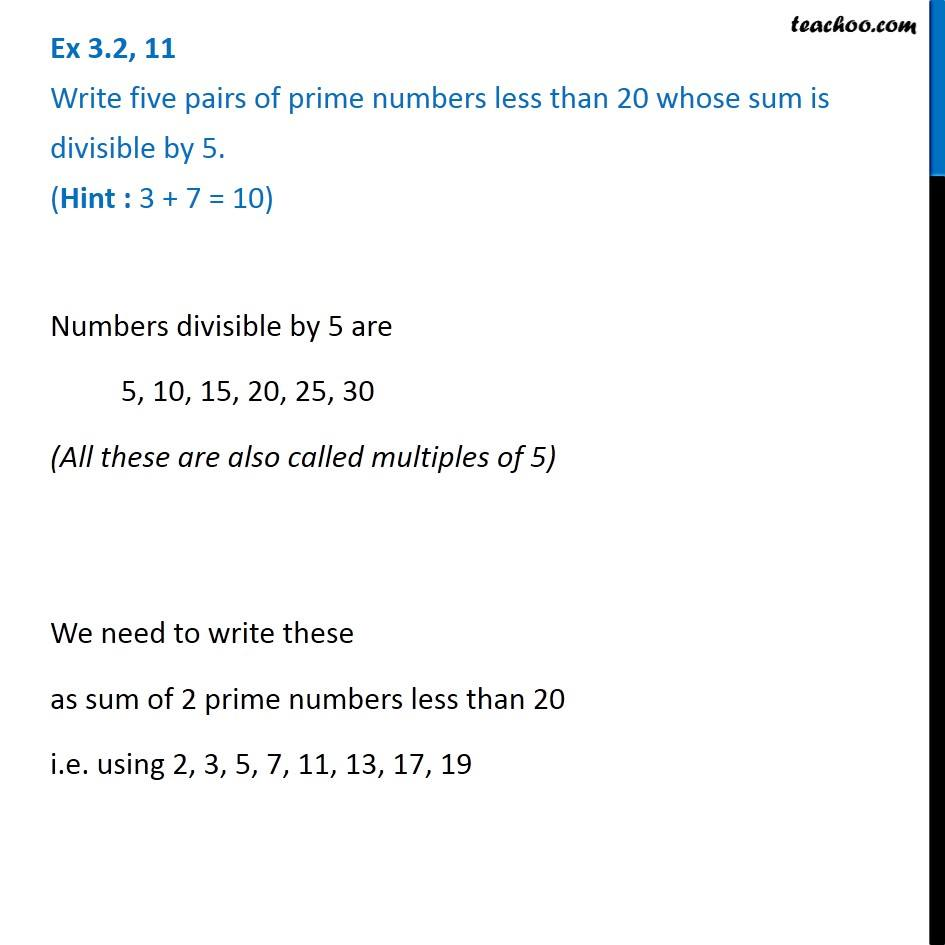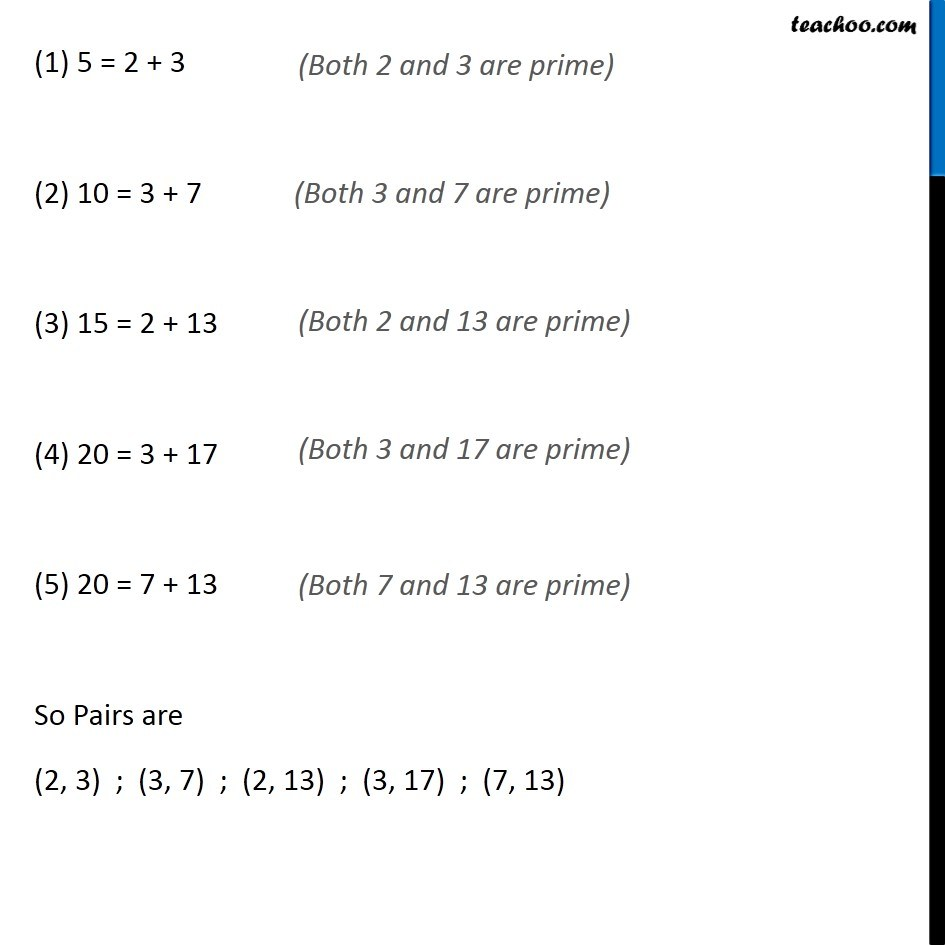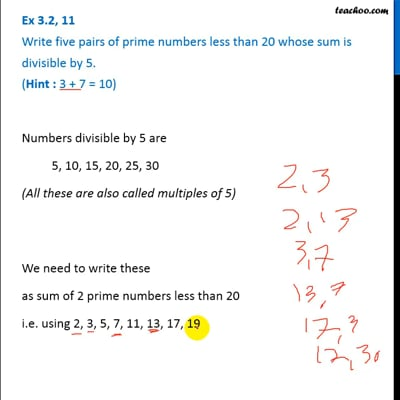Prime and Composite Numbers

Chapter 3 Class 6 Playing with Numbers
Concept wiseThis video is only available for Teachoo black users

Introducing your new favourite teacher - Teachoo Black, at only ₹83 per month

### Transcript

Ex 3.2, 11 Write five pairs of prime numbers less than 20 whose sum is divisible by 5. (Hint : 3 + 7 = 10)Numbers divisible by 5 are 5, 10, 15, 20, 25, 30 (All these are also called multiples of 5) We need to write these as sum of 2 prime numbers less than 20 i.e. using 2, 3, 5, 7, 11, 13, 17, 19 (1) 5 = 2 + 3 (2) 10 = 3 + 7 (3) 15 = 2 + 13 (4) 20 = 3 + 17 (5) 20 = 7 + 13 So Pairs are (2, 3) ; (3, 7) ; (2, 13) ; (3, 17) ; (7, 13) (Both 2 and 3 are prime) (Both 3 and 7 are prime) (Both 2 and 13 are prime) (Both 3 and 17 are prime)